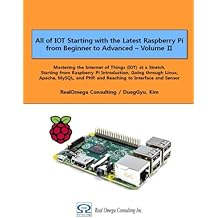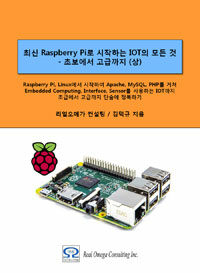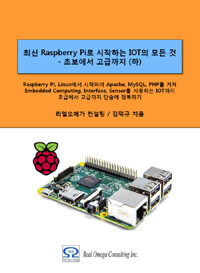# Raspberry Pi_Eng_10.2.2 Environment Variable

#### Published Book on AmazonAll of IOT Starting with the Latest Raspberry Pi from Beginner to Advanced – Volume 1All of IOT Starting with the Latest Raspberry Pi from Beginner to Advanced – Volume 2

#### 출판된 한글판 도서최신 라즈베리파이(Raspberry Pi)로 시작하는 사물인터넷(IOT)의 모든 것 – 초보에서 고급까지 (상)최신 라즈베리파이(Raspberry Pi)로 시작하는 사물인터넷(IOT)의 모든 것 – 초보에서 고급까지 (하)

### 10.2.2 Environment Variable

When the system is booted and a particular user logs on, the user is presented with a predefined screen and if the user issues a certain command, the command is processed in a predefined manner. At this time, the user confirms the contents defined in advance and determines the concrete processing method. When making important decisions that is necessary for the system to works, the system refers to a number of predefined data. Among these data, the important variables that the system frequently uses are stored in a special place, which is called an environment variable.

This environment variable stores only the most important information among many information that is necessary to operate the system. It is stored in the memory of the system rather than being stored in a specific file, so that the information can be used immediately if necessary.

#### 10.2.2.1"env" Command – Confirm Environment Variable

Then, let's see what information is stored in environment variables. The command to use at this time is the "env" command.

[Command Format]

 env  [option]

[Command Overview]

This checks the contents of the information stored in the environment variable.

User privilege          -- Normal user.

[Detail Description]

If no option is specified, the current value is displayed.

[Main Option]

 -u --unset=NAME remove variable from the environment

[Used Example]

First, let's see what the current environment variables are. Execute the command as follows.

 pi@raspberrypi ~ \$ env TERM=xterm SHELL=/bin/bash SSH_CLIENT=192.168.1.230 49229 22 SSH_TTY=/dev/pts/0 USER=pi LS_COLORS=rs=0:di=01;34:ln=01;36:mh=00:pi=40;33:so=01;35:do=01;35:bd=40;33;01:cd=40;33;01:or=40;31;01:su=37;41:sg=30;43:ca=30;41:tw=30;42:ow=34;42:st=37;44:ex=01;32:*.tar=01;31:*.tgz=01;31:*.arj=01;31:*.taz=01;31:*.lzh=01;31:*.lzma=01;31:*.tlz=01;31:*.txz=01;31:*.zip=01;31:*.z=01;31:*.Z=01;31:*.dz=01;31:*.gz=01;31:*.lz=01;31:*.xz=01;31:*.bz2=01;31:*.bz=01;31:*.tbz=01;31:*.tbz2=01;31:*.tz=01;31:*.deb=01;31:*.rpm=01;31:*.jar=01;31:*.war=01;31:*.ear=01;31:*.sar=01;31:*.rar=01;31:*.ace=01;31:*.zoo=01;31:*.cpio=01;31:*.7z=01;31:*.rz=01;31:*.jpg=01;35:*.jpeg=01;35:*.gif=01;35:*.bmp=01;35:*.pbm=01;35:*.pgm=01;35:*.ppm=01;35:*.tga=01;35:*.xbm=01;35:*.xpm=01;35:*.tif=01;35:*.tiff=01;35:*.png=01;35:*.svg=01;35:*.svgz=01;35:*.mng=01;35:*.pcx=01;35:*.mov=01;35:*.mpg=01;35:*.mpeg=01;35:*.m2v=01;35:*.mkv=01;35:*.webm=01;35:*.ogm=01;35:*.mp4=01;35:*.m4v=01;35:*.mp4v=01;35:*.vob=01;35:*.qt=01;35:*.nuv=01;35:*.wmv=01;35:*.asf=01;35:*.rm=01;35:*.rmvb=01;35:*.flc=01;35:*.avi=01;35:*.fli=01;35:*.flv=01;35:*.gl=01;35:*.dl=01;35:*.xcf=01;35:*.xwd=01;35:*.yuv=01;35:*.cgm=01;35:*.emf=01;35:*.axv=01;35:*.anx=01;35:*.ogv=01;35:*.ogx=01;35:*.aac=00;36:*.au=00;36:*.flac=00;36:*.mid=00;36:*.midi=00;36:*.mka=00;36:*.mp3=00;36:*.mpc=00;36:*.ogg=00;36:*.ra=00;36:*.wav=00;36:*.axa=00;36:*.oga=00;36:*.spx=00;36:*.xspf=00;36: MAIL=/var/mail/pi PATH=/usr/local/sbin:/usr/local/bin:/usr/sbin:/usr/bin:/sbin:/bin:/usr/local/games:/usr/games PWD=/home/pi LANG=en_US.UTF-8 SHLVL=1 HOME=/home/pi LOGNAME=pi SSH_CONNECTION=192.168.1.230 49229 192.168.1.202 22 _=/usr/bin/env

Environment variables contain many items. We will try to understand some of them:

SHELL=/bin/bash

It means the currently used Shell program.

USER=pi

It refers to the user account currently logged on.

PATH=/usr/local/sbin:/usr/local/bin:/usr/sbin:/usr/bin:/sbin:/bin:/usr/local/games:~~~

It refers to the order in which the program is searched when executing the Shell command. ":" is a symbol that separates each path. It start search from the left and to the right.

PWD=/home/pi

It indicates the current directory location.

HOME=/home/pi

It means the home directory of the currently login user

There is another way to check what the current environment variables are. The environment variables that the system has are freely available in the form of <\$environment variables> in other commands.

Here, we will use the "echo" command to find out the value of the PATH environment variable.

 pi@raspberrypi ~ \$ echo \$PATH /usr/local/sbin:/usr/local/bin:/usr/sbin:/usr/bin:/sbin:/bin:/usr/local/games:/usr/games

#### 10.2.2.2"export" Command – Define Environment Variable

You can define additional environment variables or modify the values for existing environment variables. The command to modify the environment variables is the "export" command.

[Command Format]

 export  [option]   environment-variable="value"

[Command Overview]

This defines environment variables newly or changes the content of environment variables.

User privilege          -- Normal user.

[Detail Description]

The name of the environment variable is usually defined in uppercase letters.

A defined environment variable can be used in your own Shell or child Shell.

The values stored in the environment variables are not stored in the file but are stored in memory, so they are not stored permanently and disappear when the system is down. Therefore, changing an environment variable does not mean that the value is kept forever, and the change only has a temporary effect.

Effective scope - Changes made in the Shell are valid only in the Shell, not in other Shells. Even if you change from a specific Terminal, it remains unchanged when you open another Terminal screen.

[Main Option]

[Used Example]

We will add "/opt/testbin/" to the value of the current PATH environment variable. Make the following changes and check the contents again. PATH value has changed as expected.

 pi@raspberrypi ~ \$ echo \$PATH /usr/local/sbin:/usr/local/bin:/usr/sbin:/usr/bin:/sbin:/bin:/usr/local/games:/usr/games pi@raspberrypi ~ \$ export PATH="\$PATH:/opt/testbin" pi@raspberrypi ~ \$ echo \$PATH /usr/local/sbin:/usr/local/bin:/usr/sbin:/usr/bin:/sbin:/bin:/usr/local/games:/usr/games:/opt/testbin

The following is an example of a newly defined environment variable that is not defined in the system but is to be used separately.

 pi@raspberrypi ~ \$ env pi@raspberrypi ~ \$ export TESTENV="\$HOME/Script" pi@raspberrypi ~ \$ env pi@raspberrypi ~ \$ echo \$TESTENV

#### 10.2.2.3Fixing Value of Environment Variable Permanently

Normally, the value of the environment variable is lost when the system is shut down, and the old value can not be used when the system is newly started. If you want to permanently fix the value set in the environment variable, you can use profile.

You can use the following profile provided by the system:

System profile         -- /etc/profile file            -- It applies to all users.

User profile            -- ~/.profile file             -- It applies to a specific user.